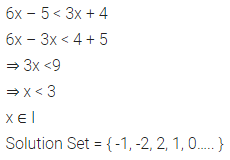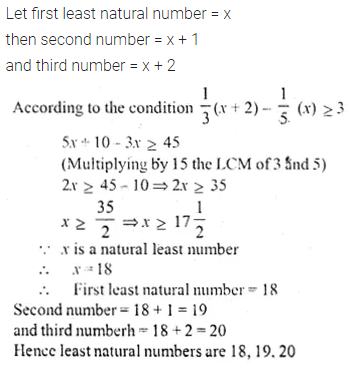# ML Aggarwal Class 10 Solutions for ICSE Maths Chapter 4 Linear Inequations Chapter Test

## ML Aggarwal Class 10 Solutions for ICSE Maths Chapter 4 Linear Inequations Chapter Test

ML Aggarwal Class 10 Solutions for ICSE Maths Chapter 4 Linear Inequations Chapter Test

Question 1.
Solve the inequation : 5x – 2 ≤ 3(3 – x) where x ∈ { – 2, – 1, 0, 1, 2, 3, 4}. Also represent its solution on the number line.
Solution:Question 2.
Solve the inequations :
6x – 5 < 3x + 4, x ∈ I.
Solution:Question 3.
Find the solution set of the inequation
x + 5 < 2 x + 3 ; x ∈ R
Graph the solution set on the number line.
Solution:Question 4.
If x ∈ R (real numbers) and -1 < 3 – 2x ≤ 7, find solution set and represent it on a number line.
Solution:Question 5.
Solve the inequation :
$$\frac { 5x+1 }{ 7 } -4\left( \frac { x }{ 7 } +\frac { 2 }{ 5 } \right) \le 1\frac { 3 }{ 5 } +\frac { 3x-1 }{ 7 } ,x\in R$$
Solution:Question 6.
Find the range of values of a, which satisfy 7 ≤ -4x + 2 < 12, x ∈ R. Graph these values of an on the real number line.
Solution:Question 7.
If x ∈ R, solve $$2x-3\ge x+\frac { 1-x }{ 3 } >\frac { 2 }{ 5 } x$$
Solution:Question 8.
Find positive integers which are such that if 6 is subtracted from five times the integer then the resulting number cannot be greater than four times the integer.
Solution:Question 9.
Find three smallest consecutive natural numbers such that the difference between one-third of the largest and one-fifth of the smallest is at least 3.
Solution: